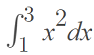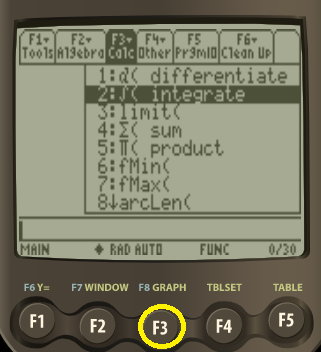# TI 89 Integral: Step by Step Examples

TI 89 Integral Contents:

## TI 89 Integral Function for Definite Integrals

General steps for finding the value of a definite integral on the TI-89:

1. Press the F3 button,
2. Press the “2” button (or scroll down to 2: ∫ ( integrate then press the “ENTER” button.)
3. Type in your function in the form
∫ (f(x), x, a, b).

See below for a specific example of finding a TI 89 integral.

## Example #1: Definite Integral on the TI 89

Example question:Evaluate:To put this in English, the question is asking you to find the definite integral of f(x) = x2 on the closed interval [1, 3] (1, 3 are called the bounds of integration).

Step 1: Press the F3 button:Step 2: Press “2”:Step 3: Type in your function. For this example, that’s x2, so type in
x ^ 2Your screen should look like this:Step 4: Type a comma, then type the letter x .

Step 5: Type a comma again, then type your bounds of integration. The bounds of integration for this example are 1, 3 so type in
1,3
(don’t forget to type in another comma to separate the bounds!).

Step 6: Type a closing parenthesis. Your formula should look like this:Step 7: Press ENTER. The solution is 8.66667.## TI 89 Integral: General Instructions for Antiderivatives

Indefinite integrals don’t have any bounds of integration. Follow the above steps to find indefinite integrals, omitting Step 5 (i.e. don’t type a,b).

Example question: What is the antiderivative of f(x) = 3x5?

Step 1: Press the F3 button..

Step 2: Press “2”.

Step 3: Type in your function. For this example, that’s 3x5, so type in
3 x ^ 5

Step 4: Type a comma, then type the letter x .

Step 5: Type a closing parenthesis.

Step 7: Press ENTER. The solution the calculator gives is x6/2.